# Valency and Valence Electrons: Definition, Examples and Relation

• Last Updated : 24 Jan, 2022

Element atoms have a natural capability to bond with other element atoms. A molecule is formed when two or more atoms of the same or different elements unite to form a stable structure. A molecule of sodium chloride is formed when an atom of sodium and a molecule of chlorine unite, while a molecule of hydrogen fluoride is formed when an atom of hydrogen and a molecule of fluorine combine. Each atom has some capability for mixing with other atoms. Valency refers to an element’s ability to combine its constituent atoms. Hydrogen and chlorine are believed to have the same valency. The valency of other elements is determined by comparing them to hydrogen or chlorine.

Atoms are made up of electrons, protons and neutrons. Generally, protons and neutrons lie at the center of an atom called the nucleus and electrons revolve around the nucleus in a fixed path called orbits or shells.

The shells are named ‘K’, ‘L’, ‘M’, ‘N’ and so on. The innermost shell is the ‘K’ shell which contains up to 2 electrons, followed by the ‘L’ shell which contains up to 8 electrons followed by the ‘M’ shell which contains up to 18 electrons and then the ‘N’ shell which contains up to 32 electrons. The different types of atoms come together and form elements.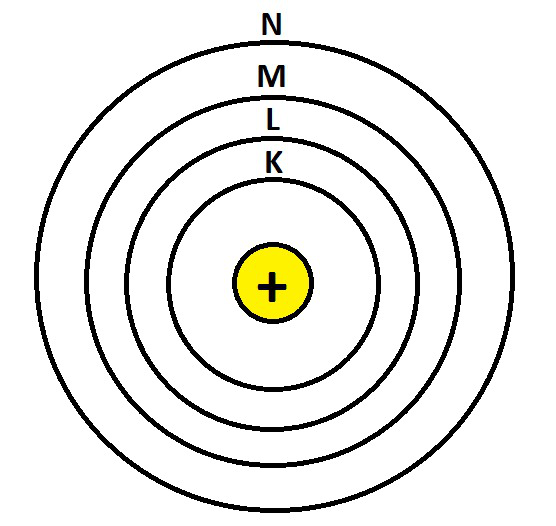Structure of Atom

### What is Valence electrons?

The electrons in the outermost shell around an atomic nucleus are known as valence electrons. Valence electrons are important because they provide detailed information on an element’s chemical characteristics, such as whether it is electronegative or electropositive in nature, or the bond order of a chemical compound, which indicates the number of bonds that may be formed between two atoms.

Thus, valence electrons are defined as,

The number of electrons present in the outermost shell of an element that takes part in chemical bonding is called valence electrons.

For example, Lithium (Li) has atomic number equal to 3. Its electronic configuration is K = 2 and L = 1, this implies, K subshell is fully filled while L subshell i.e. the outermost shell has only 1 electron, which is its valence electron as shown in the below diagram: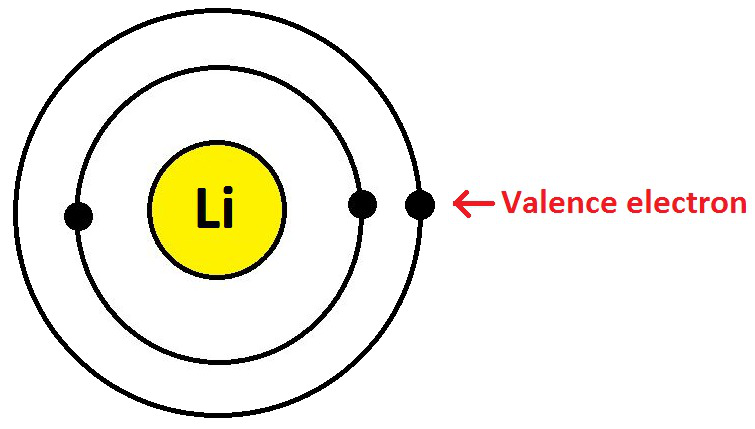Valence Electron in a Lithium atom

Changes in the atomic structure are confined to the electrons in the outermost shell, i.e. valence electrons, regardless of the type of chemical interaction between atoms, whether it an ionic, covalent, or metallic bond.

A valence electron is an electron that is attached to an atom and may be used to establish a chemical interaction; in a single covalent bond, both atoms contribute one valence electron to form a shared pair. The number of valence electrons can affect the chemical characteristics of an element as well as its ability to interact with other elements:

• A valence electron in a main group element can only be in the outermost electron shell.
• Chemically, an atom with a closed shell of valence electrons is inert. Because the additional valence electrons are quickly removed to create a positive ion, an atom with one or two valence electrons more than a closed shell is extremely reactive.
• Because of a tendency to either obtain the missing valence electrons (creating a negative ion) or share valence electrons, an atom with one or two valence electrons fewer than a closed shell is also extremely reactive (thereby forming a covalent bond).
• A valence electron, like an electron in an inner shell, may receive or release energy in the form of a photon. Atomic excitation occurs when an electron gains enough energy to migrate (jump) to an outer shell.
• Ionization occurs when an electron breaks away from its associated atom’s valence shell, resulting in the formation of a positive ion. When an electron loses energy (and so emits a photon), it might migrate to an inner shell that isn’t completely occupied.

Determination of Valence Electrons in an Atom

Although the number of shells rises as we travel down a group, the number of valence electrons remains constant.

While the number of valence electrons increases by one over time, the number of shells remains constant. The number of shells around an element’s nucleus is indicated by the period number (row number).

### What is Valency?

The valency of an atom is equal to the number of valence electrons that this atom can gain or lose during chemical reactions. Or in other words:

The amount of hydrogen atoms, chlorine atoms, or double the number of oxygen atoms that one atom of an element may combine with is referred to as its valency.

The valency of an element refers to the number of valence electrons in that element that participate in chemical processes. A chemical compound is created when two or more components are combined in a certain mass proportion. A stable compound is formed when one atom of one element unites with a specific number of atoms of another element. All components have different capabilities for mixing.

Valency of Metals

A metal is an element with one, two, or three electrons in its valence shell (except hydrogen and helium, which are non-metals). In chemical processes, metals tend to shed their valence electrons and complete their octet. Therefore,

Valency of metallic element = Number of electrons in its valence shell

Elements from Group 1, 2 & 13 of the periodic table have valencies 1, 2, 3 respectively which is similar to the electron configuration in their last shell.

For Example:

• Sodium (Na)

The Electronic configuration of Na is 11 (K = 2; L = 8; M = 1). They have 1 electron in their outermost shell. So, the valency of Sodium is 1 as shown below: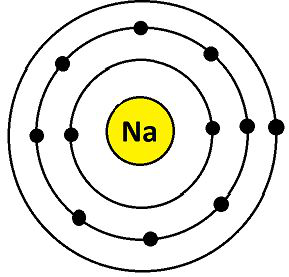Valency of Sodium

• Magnesium (Mg)

The Electronic configuration of Mg is 12 (K = 2; L = 8; M = 2). They have 2 electrons in their outermost shell. So, the valency of Magnesium is 2 as show below: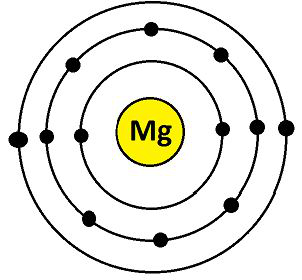Valency of Magnesium

• Aluminium (Al)

The Electronic configuration of Al is 13 (K = 2; L = 8; M= 3). They have 3 electrons in their outermost shell. So, the valency of Aluminium is 3 as shown below: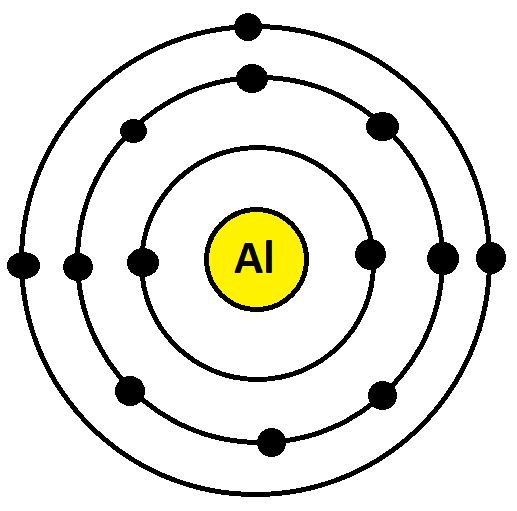Valency of Aluminium

Valency of Non-Metals

If an element’s valence shell has 5 or 6 or 7 or 8 electrons, it is classified as a non-metal. To complete its octet, a non-metal requires 3 or 2 or 1 or 0 electrons. As a result, a non-metallic element’s valency = 8 – number of electrons in its outermost shell.

Elements from Groups 15, 16 & 17 of the periodic table have 5, 6, 7 electrons respectively in their last shell so the valency of non-metals is decided by finding the number of electrons required to complete its octet configuration.

For example:

• Nitrogen (N)

The Electronic configuration of N is 7 (K = 2; L = 5; M= 0). They have 5 electrons in their outermost shell. So, the valency of Nitrogen is: 8 – 5 = 3 as shown below: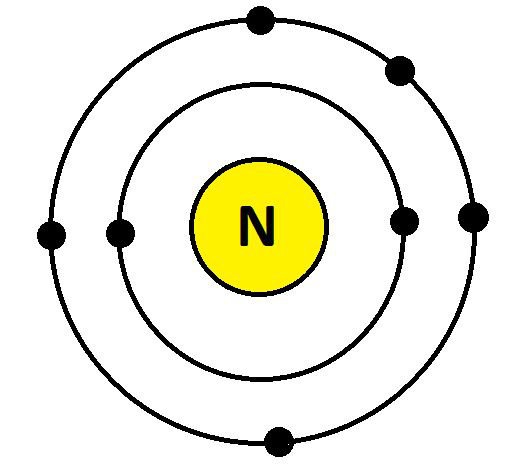Valency of Nitrogen

• Oxygen (O)

The Electronic configuration of O is 8 (K = 2; L = 6; M= 0), They have 6 electrons in their outermost shell. So, the valency of Oxygen is : 8 – 6 = 2 as shown below: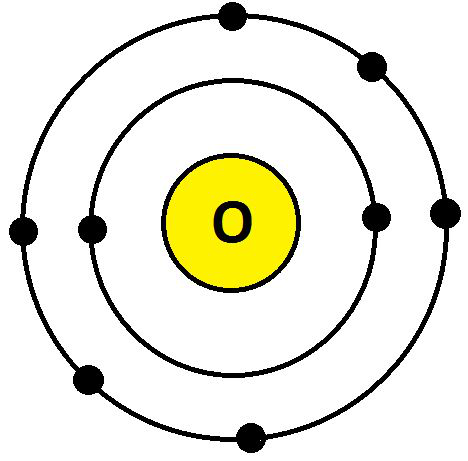Valency of Oxygen

• Fluorine (F)

The Electronic configuration of F is 9 (K = 2; L = 7; M= 0). They have 7 electrons in their outermost shell. So, the valency of Fluorine is: 8 – 7 = 1 as shown below: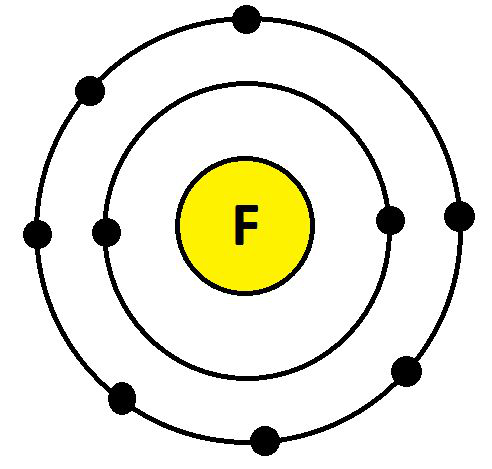Valency of Fluorine

Valency of Nobel gases

Group 18 elements of the periodic table are called noble gases because they do not react at all as they have the most stable electronic configuration due to having the maximum number of valence electrons in its outer shell. The valency is zero as every noble gas’s outermost shell is filled hence they do not lose or gain any electron.

For Example:

• Neon (Ne)

The electronic configuration of Ne is 10 (K = 2; L = 8; M= 0). They have 8 electrons in their outermost shell. So, the valency of Neon is : 8 – 8 = 0.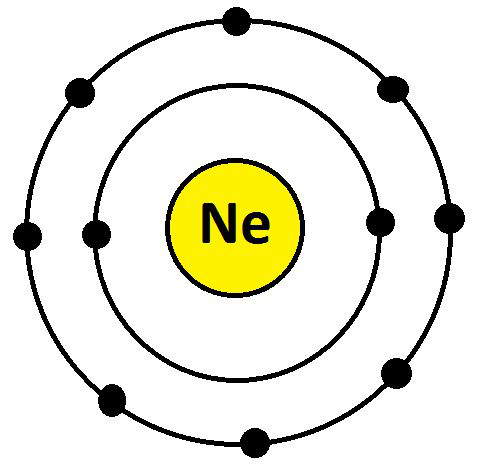Valency of Neon

### Relation Between Valency and Valence Electrons

Valency explains the bond formation of atoms. Whereas, valence electrons are related to elemental characters. Valency is only a concept or idea and doesn’t involve transmission of electrons. Whereas, valence electron involves transmission of electrons in the formation of bonds. Both valency and valence electrons are applied for any chemical element.

Since, only the valence electrons (outermost electrons) participate in chemical bonding, an element’s valency is determined by the number of valence electrons (outermost electrons) in its atom. The amount of valence electrons in an element’s atom or the number of electrons necessary to complete eight electrons in the valence shell determines the element’s valency. Sodium, for example, contains one valence electron and thus a valency of one. As a result, the valency of sodium is equal to the amount of valence electrons in its atom. A metal element’s valency is equal to the number of valence electrons in its atom in general.

Valency of a metal = Number of valence electrons in its atom

A valence electron, like an electron in an inner shell, may receive or release energy in the form of a photon. Atomic excitation occurs when an electron gains enough energy to migrate (jump) to an outer shell. Ionization occurs when an electron breaks away from its associated atom’s valence shell, resulting in the formation of a positive ion. When an electron loses energy (and so emits a photon), it might migrate to an inner shell that isn’t completely occupied.

Valency of a non-metal = 8 – Number of valence electrons in its atom

The valency of hydrogen is the one exception to this rule. Hydrogen’s valency is equal to the number of valence electrons, which is one (though hydrogen is a non-metal element).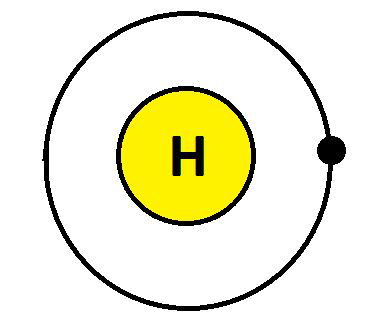Valency of Hydrogen

### Sample Questions

Question 1: Name the electrons of an atom that are involved in bond formation?

Only valence electrons of an atom are involved in bond formation.

Question 2: What is the electron distribution of the Chlorine atom? How many electrons are there in the last shell?

Since, the atomic number of a Chlorine atom (Cl) is 17.

Therefore, the electronic distribution of Cl: K = 2, L = 8, M = 7

And the last shell has 7 electrons.

Question 3: What is the electronic configuration of a positively charged sodium ion (Na+)?

We know that, Atomic number of Na is 11

Since, Atomic number = Number of protons = 11

Also, in a neutral atom the number of protons = number of electrons = 11

So, Number of electrons in (Na+) ion = 11 – 1 = 10

Thus, the electronic configuration of (Na+) ion is K = 2, L = 8.

Question 4: An element ‘E’ contains 7 electrons in the ‘M’ shell as valence electrons. What is the atomic number of ‘E’? And also check whether ‘E’ is a metal or non-metal.

As ‘E’ has 7 electrons in the ‘M’ shell as valence electrons, the electronic configuration of ‘E’ is K = 2, L = 8, M = 7.

This implies, the atomic number is 17.

Also, we know that elements of groups 15, 16, 17 along with Hydrogen is non – metal.

So, ‘E’ is a non – metal.

Question 5: The atomic number of lithium is 3. Its mass number is 7. How many protons and neutrons are present in a lithium atom?

We know that,

Number of neutrons = Mass number – Atomic number

So, Number of neutrons = 7 – 3 = 4

And, Number of protons = Atomic number

Hence, Number of protons = 3.

Question 6: Why Helium, Neon and Argon have zero valency?